# HP 50g Derivatives, Integrals, and Summations Tutorial

Arguably of the best reasons for getting a calculator with a CAS (computer algebra system) is to solve derivatives, integrals, and summations symbolically. Here’s how to do it with the HP 50g.

NOTE: This (and all of my other) tutorial assumes that the calculator is in RPN mode. If you are not using RPN mode, take the time to learn it and you will be very glad you did!

### Derivatives

To take the derivative of an expression where the independent variable is ‘X’, first enter the expression onto the stack.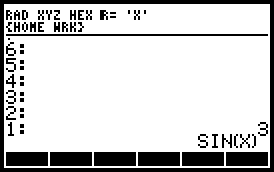Next press [LSHIFT], then [CALC] to open up the calculus menu. Select {DERVX}, which stands for “derivative with respect to X”, and press the {OK} soft button.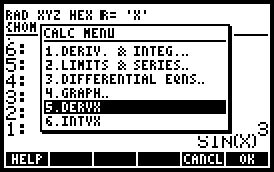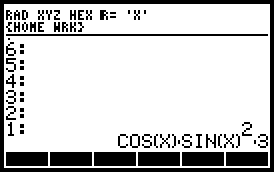### Indefinite Integrals

To take the indefinite integral of an expression where the independent variable is ‘X’, first enter the expression onto the stack.Next press [LSHIFT], then [CALC] to open up the calculus menu. Select {INTVX}, which stands for “integral with respect to X”, and press the {OK} soft button.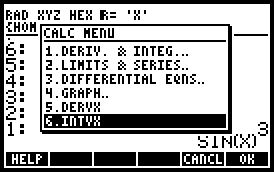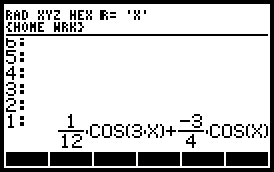### Definite Integrals

To take the definite integral of an expression, open up the equation writer by pressing [RSHIFT] then [EQW]. To enter an integral press [RSHIFT] and then the integral button (same as the [TAN] button). Your calculator should look like this: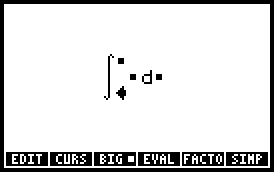Enter values for all the “dots” by entering the desired values and then pressing the [RIGHT ARROW] button to move to the next location. A possible input could look like this.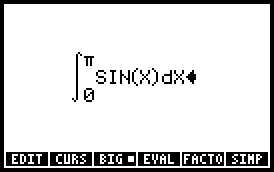It should be noted that in the equation writer the calculator can integrate with respect to any variable, just make sure to put the desired variable in the differential. Once the integral is set up correctly, press [ENTER] to output the expression onto the stack.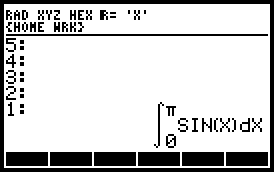To evaluate the expression, press [EVAL]. Hopefully your calculator shows this!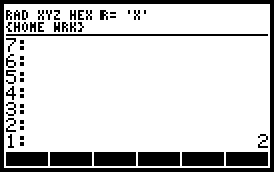### Summations

Finding the value of a summation is similar to solving a definite integral. Open up the equation writer by pressing [RSHIFT] then [EQW]. To enter an summation press [RSHIFT] and then the sigma button (same as the [SIN] button).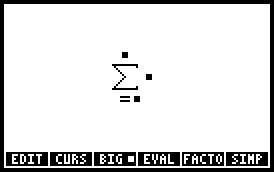Enter values for all the “dots” by entering the desired values and then pressing the [RIGHT ARROW] button to move to the next location. A possible input could look like this.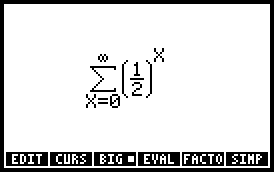Press [ENTER] to output the expression onto the stack.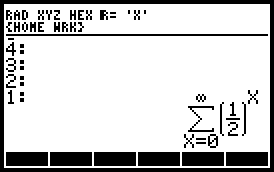To evaluate the expression, press [EVAL]. Hopefully your calculator shows this!